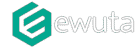# What We Need to Know About Derivation and Integration

We all have different choices and likeness. Some people like chemistry and others like math and physics. If you are from the second category, then this is for you. Math and physics are now part of our daily routine including study.

Whenever we talk about math there are two types of concepts that arise into our mind. The first one is Derivation and the second one is Integration. Derivation and integration are two important parts of maths and physics. They fall under concepts that are major for calculation.

Here, we will discuss the facts that we need to know about derivation and integration?

The derivation is defined as the origination of some mathematical algorithm data by understanding any physical phenomena. Have you ever noticed the concept which physics and mathematics are providing to us?

These two subjects tell us about the logic behind every concept and phenomena that are used to understand that logic. Moreover, it increases our understanding to an exceptional level.

• The derivation is used to study only the small changes within a quantity concerning its unit.
• Its example included: rate of change of speed concerning its velocity.

Integration is used to add data which is small and discrete. This data cannot be added in a single value. Therefore the integration process is helpful to add the as a whole.

• Its example includes: if you are finding an area between curves for large-scale industries then you can use the integration method.

## What is derivation?

A derivative is a rate of change that resembles the slope of a graph. Geometrically it can be seen in the form of slope of a graph. If we talk about derivation in context to physics, there are the following concepts:

• If we said that acceleration is the rate of change of velocity then we will see that mathematical acceleration is the derivative of the velocity.
• when the velocity is the rate of change of position then velocity is the derivative of position.
• Likewise, if the net force is the rate of change of momentum then the derivative for an object’s momentum will tell you about the net force on the object.

We can calculate derivatives in the same way as we calculate integration, the process of calculations is slightly different but the concepts remain the same.

## Applications of derivations:

Derivations have several important applications not only in physics or math but also in our daily life. It also has its use in other related fields like science and engineering. If we talk about only math there are multiple impacts that derivations have:

• It is used to rate the change of quantity
• It is used for linear approximations
• It is used to create tangent on a curve
• It is used for Newton’s method
• It is used for an equation to increase and decrease a particular function.

## What is integration?

Integration is the process that is used to find out the central point, volume, and areas. Integration is a method which is used to find out definite integrals in a context. It has a unique set of instructions to find out the area for the curve.

### For example:

If you are finding a derivative Dg(x) which has function g(x), then it will be equal to the f(x). This may be indicated by a sign, known as an integral sign  “∫,” as in ∫f(x). This is called the indefinite integral of the function.

## Application for integration:

There are multiple applications on integration that are present in math. This included the following:

• It is used to Determine volume by slicing method
• It is used to find out the areas between the curves
• It is used to find out the volume
• It is used to find out moments and center of mass

### Derivation:

• It is used to calculate the gradient of a curve.
• It is the direct opposite of the integration.
• It uses the division method for calculations.
• It is used to calculate the speed of the function.
• Its real-life example included calculating instantaneous velocity.

### Integration:

• It is used to calculate the area under the curve.
• It is directly opposite to the derivation.
• It uses the addition method for calculations.
• It calculates the distance that is covered by function.
• It is used in real life to calculate the central point, areas, and volume.

#### Conclusion:

There we have discussed the two major concepts which have a revolutionary role in physics and math. These concepts are Derivation and Integration. Both of these relate to each other but are opposite to each other.

Here we have described the facts that we need to know about derivation and integration? These are some important points that you need to consider and keep them in mind before their use.